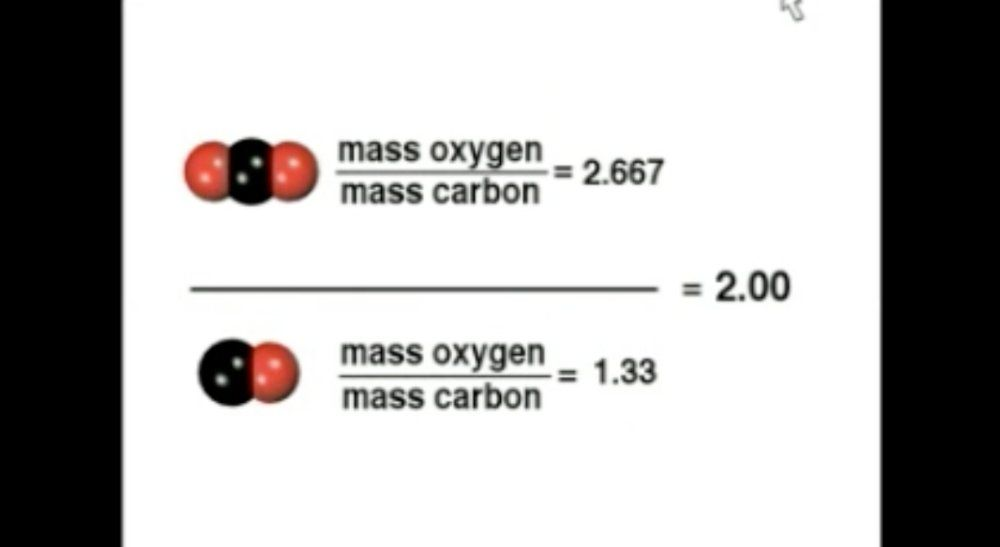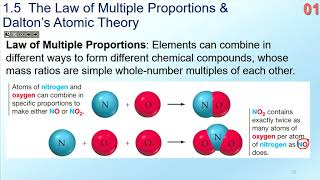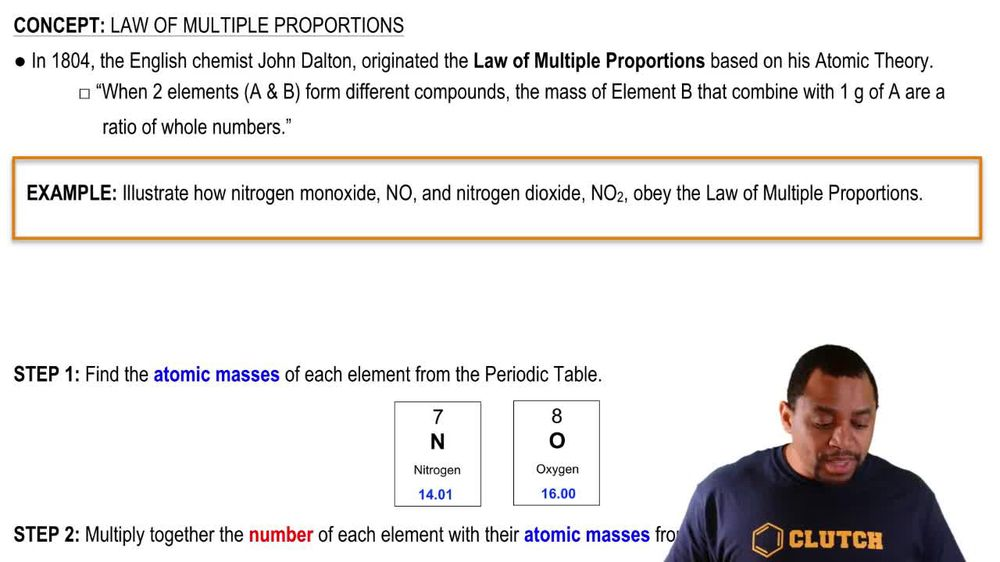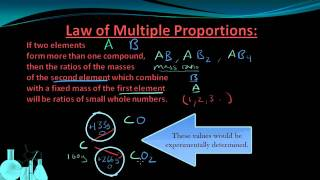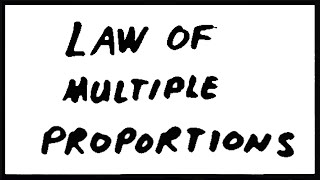Start typing, then use the up and down arrows to select an option from the list.
1. 2. Atoms & Elements2. Law of Multiple Proportions
Problem

# In borane, one part hydrogen combines with 3.6 parts boron by mass. A compound containing only hydrogen and boron contains 6.0 g of hydrogen and 43.2 g of boron. Could this compound be borane? If it is not borane, show that the law of multiple proportions is followed for borane and this other substance.

Relevant Solution8m
Play a video:
hi everyone for this problem we're told that phosphorus try bromide and phosphorus pinto bromide are compounds made from phosphorus and bro. Mean When decomposed an amount of phosphorus Triboro meid gives 1.4463 g of phosphorus and 11.1946 g of bra mm. And an amount of phosphorus Penta bromide gives .2766 g of phosphorus and 3.5677 g of browning. We need to compute the ratio of grooming to phosphorus for both of our compounds and secondly, determine if it follows the law of multiple proportions. So we have two parts of this problem and let's go ahead and get started with the first part. So well compute the ratio of grooming to phosphorus for both of our compounds. For phosphorus try bromide that's represented as P B. R three. And our ratio of bro mean to phosphorus is equal to 321. So we have three borough means for everyone phosphorus. Now on the other hand are phosphorus Penta bromide is represented as PB. R five and our ratio of bro mean it's a phosphorus is five to one. So that's the first part. This is our ratio of bromine to phosphorus for both of our compounds. Now we need to determine if it follows the law of multiple proportions, what the law of multiple proportions tells us that when we have two elements that combine with each other in this case phosphorus and bro mean And they form two or more compounds here we have two compounds. The ratio is a simple whole number. And so in order for us to figure out if this follows the law of multiple proportions. The first thing we need to do is convert each element to moles from the given mass. And then we can calculate the ratio to see if we have a simple whole number. So this is what I mean for phosphorus Penta bromide, we need to convert our grams of phosphorus 1. 463 g of phosphorus two moles. And we can do this using the molar mass of phosphorus and one mall of phosphorus we have 30 point 97 g of phosphorus. Our grams of phosphorus cancel. And we're left with 0. moles of phosphorus. Now let's do the same thing with our browning. We're told that we have 11. grams of browning. And we need to go from grams to moles. So we can do that using the molar mass and one mole of bro mean We have 79 . g of bro mean our units cancel and we have zero point moles of browning. So now let's calculate this ratio of moles of grooming two moles of phosphorus using the molds that we just calculated. So we have 0. 01 malls of browning and we have 0. moles of phosphorus, This ratio gives us a ratio of three. So this follows the law of multiple proportions because the ratio of grooming per mole of phosphorus is a whole number. So for the first part we calculated the ratio to be 3-1 and it does follow the law of multiple proportions because once we calculated our ratio we got a simple whole number. So now we need to do the same thing for phosphorus pinto bromide to see if it also follows the law of multiple proportions. And we're told that we have 0. grams of phosphorus and one mole of phosphorus We have 97 g of phosphorus. So this gives us zero point moles of phosphorus. And we're told that we have three point 5677 g of bro mean and one more of bro mean we have 79.90 g of roaming. This gives us 0. moles of bro mean. So now once we calculate our ratio We have zero malls of bro mean over 0.00893 moles of phosphorus. And our ratio is equal to five. And this also follows the law of multiple proportions because the ratio of bromine per mole of phosphorus is a whole number. So for the second part We had a ratio of 5- and then we just proved why it follows the law of multiple proportions. So that is both parts of this problem and that is it. That's the end of this problem. I hope this was helpful.